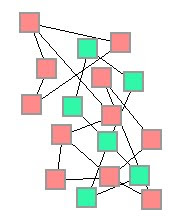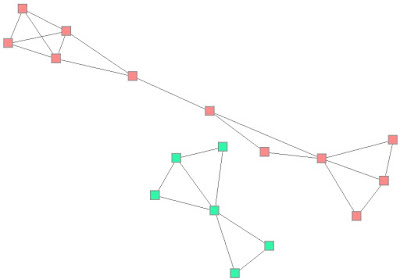# Force-Directed graph layout approximation part II

In this article I'll describe the different calculations that we have to do in Repulsive and Attraction methods to calculate the position of the different nodes. As I published yesterday in part I, I'll focus on the Hooke's and Coulomb's laws and the integration inside the platform.
• Hooke's Law (attractive force):The functionality here is very simple, we need to think that every edge represents a spring, and when 2 nodes that are connected try to move in opposite direction (by a force of repulsion), the spring tend to be deformed by that force. When this happens (the spring is being forced to move) , it returns to the initial state of equilibrium. For this, we need to create a model to generate this behaviour. I've found several examples on the Internet, like in johngrindall.com (Pendulum simulation ), for example in youtube we can find very good videos explaining the hooke's law:

Anyway, in order to do this, I've had to change a little the TBox Class, now I need this new information:Now the Tbox class manages information concerned to the Node class (in this image is all mixed together, and the code is still in refactoring process). However, for calculating the layout we need to know the Coulomb's force, the Hooke's force, the mass and the Speed. Notice that every one of this properties is a TForce class, this class lets me work with extended values in a pleasantly way.

To accomplish this functionality I've made a model in Delphi that looks like this:

`procedure AttractiveForce()var i, j : integer; node, node2 : TBox; radius, Hooke, vector : Extended; offset : TPointR;begin for i := 0 to Boxlist.Count - 1 do begin   node := Boxlist.items[i];   node.HookeForce.setVal(0, 0);   for j := 0 to High(node.FNeighbour) do   begin     node2 := Boxlist.GetBox(node.FNeighbour[j]);     radius = distanceNodes(node, node2, offset);     if Compare(radius, 0.0, '<>') then     begin       vector := PointR(-(offset.X / radius),-(offset.Y / radius));       Hooke.X := (vector.X * sqr(radius) / (Epsilon * node.mass));       Hooke.Y := (vector.Y * sqr(radius) / (Epsilon * node.mass));     end;     node.HookeForce.sr0(node.HookeForce.r0 + Hooke.X);     node.HookeForce.sR1(node.HookeForce.r1 + Hooke.Y);     new_kinetic_energie := new_kinetic_energie + (sqr(radius) / (Epsilon * node.mass));   end; end;end;`

At the end of the post, you can watch a little video of the running of the application.
• Coulomb's Law (repulsive force)
Coulomb's law says the electrical force between two charges (q1) and (q2) is proportional to the product of the two charges divided by the distance between the two charges squared:Images from ffden-2.

For every node, we need to calculate the same as in the previous part. In each node will appear a repulsion force because every node has a positive charge. With this phenomenon all the nodes will try to spread out in different directions, but thanks to the edges modelling springs, will stop this repulsion movement applying an attraction force until they reach the equilibrium.

In the following video from derekowens, we can see a little introduction to the Coulomb's law:

The method for calculating the repulsive force for each node:

`procedure RepulsiveForce()vari, j : integer;node, node2 : TBox;radius, vector : Extended;offset : TPointR;beginfor i := 0 to Boxlist.Count - 1 dobegin  node := Boxlist.items[i];  node.CoulombForce.setVal(0, 0);  for j := 0 to Boxlist.Count - 1 do  begin      node2 := Boxlist.items[j];      if (node.id <> node2.id) then      begin          radius = distanceNodes(node, node2, offset);          if Compare(radius, 0.0, '<>') then          begin              vector := PointR(offset.X / radius,offset.Y / radius);              node.CoulombForce.sr0(node.CoulombForce.r0 + ((Epsilon * node.mass * vector.X) / radius));              node.CoulombForce.sR1(node.CoulombForce.r1 + ((Epsilon * node.mass * vector.Y) / radius));              new_kinetic_energie := new_kinetic_energie + (Epsilon * node.mass / radius);          end;      end;  end;end;end;`

After calculating the different forces the graph will reorganize itself to show a human readable graph:We can even add edges in runtime and the system will recalculate the new connections. This video shows the result of applying the algorithm to the first graph:

1.